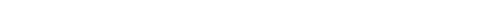## Question of the Week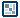Physics-Based Bounce Effect - Page 1
by kirupa  |  10 February 2009

So many of the animations you create directly or indirectly are inspired by motion in the physical world. Yet, the language of motion you use in Flash is completely different from that of the mathematical equations that describe physics. In this tutorial, let's look at how to reconcile those two worlds.

The last sentence probably sounds scary. This sounds like a flashback to physics classes which often involved contrived examples of blocks on inclines, cars sliding down the road, and things dropping from tall places. This tutorial will describe things dropping from tall places! (hey, I never said that I was original)

The following is an example of the effect I will be describing. Click on the ball to see it fall again.

[ click on the ball once it hits the ground to cause it to fall again ]

The effect you see can be created easily using a few simple eases, but instead, I am defining the motion manually using good old fashioned physics equations. As you will see, the concepts behind it may be a bit complicated. The actual code is pretty straightforward.

Physics and Falling Objects
In order to understand the code that powers the above animation, it will be helpful to learn the physics behind falling objects. Let's take a look at the following diagram:The diagram is pretty representative of the the ball falling in the animation. The key things to note about this diagram are the various labels I've given to the points of interest. The distance your ball falls is the difference between the initial position and the final position. The force that causes your ball to fall is gravity, and it is always downward. Seems simple enough.

At the end of the fall, you know where the ball is going to be. It is going to be at the bottom where the final position mark is: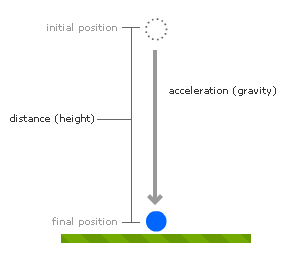The tricky part is knowing exactly where the ball is between the initial position and the final position. It's not a linear fall, so you can't evenly divide the position your ball is based on the total height. There is acceleration, so the ball moves faster through certain regions and slower through others.

Figuring this all out from scratch would be tough, but thankfully, this is something that has been well defined for a few hundred years. The following equation describes the position of your ball as it gets dropped:The a stands for acceleration, the t stands for time, and v represents velocity or speed. The position of my ball is determined by three things:

1.  My initial position (y)
2. My initial velocity (v * t)
3. Distance from the accelerated movement (.5 * a * t2)

Our initial position is the starting point of the ball drop. What about initial velocity? Well, we actually don't have an initial velocity. The value of v is 0. The reason is, at the beginning of the drop, the ball is motionless. It isn't being thrown down, so the equation can be simplified a bit as follows: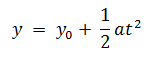This equation looks a bit nicer! Anyway, keep the last equation in mind, for that defines the structure of the code that is used to make our object fall. In the next page, we will look at the second half of the animation that concerns the physics behind objects that bounce after hitting the ground.

Onwards to the next page!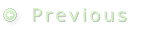1 | 2 | 3 | 4